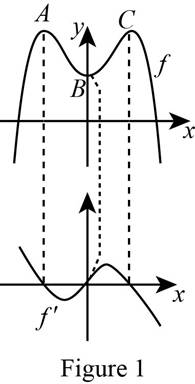# The graph of derivative of f below the graph of f.### Single Variable Calculus: Concepts...

4th Edition
James Stewart
Publisher: Cengage Learning
ISBN: 9781337687805### Single Variable Calculus: Concepts...

4th Edition
James Stewart
Publisher: Cengage Learning
ISBN: 9781337687805

#### Solutions

Chapter 2, Problem 35RE
To determine

## To sketch: The graph of derivative of f below the graph of f.

Expert Solution

### Explanation of Solution

From the given graph, it is observed that the graph of f contains horizontal tangents at three points. Let these there points be A, B and C.

Note that, the value of the derivative will be zero at the point where the function has the horizontal tangent.

Thus, the graph of f will be zero at the points A, B and C.

From the point A to left, the slope of the graph f is strictly positive which implies that the derivative graph f must have a functional value in positive.

From the point A to point B, the slope of the graph f is strictly negative which implies that the derivative graph f must have a functional value in negative.

From the point B to point C, the slope of the graph f is strictly positive which implies that the derivative graph f must have a functional value in positive.

From point C to right, the slope of the graph f is strictly negative, which implies that the derivative graph must have a functional value in negative.

Graph:

Trace the graph of f and use the above information to draw the graph of its derivative directly beneath as shown below in Figure 1.Thus, f is the required graph.

### Have a homework question?

Subscribe to bartleby learn! Ask subject matter experts 30 homework questions each month. Plus, you’ll have access to millions of step-by-step textbook answers!